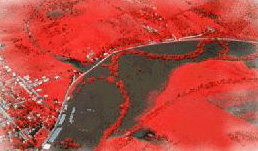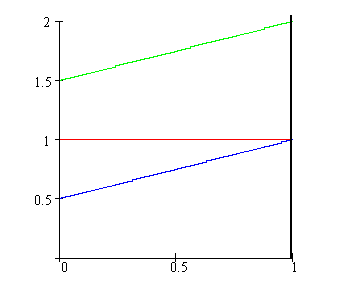Calculus on Demand at Dartmouth College Lecture 21 | Index | Lecture 23 Lecture 22## Resources

Math 3 Course Syllabus
Practice Exams

# Contents

In this lecture we define the definite integral and develop some of its immediate properties.

### Quick Question

What is the total area under the sum of the two functions f(x) = 1 and g(x) = 1/2 + x/2?### Outline

Outlines for
The Definite Integral
Properties of the Definite Integral

### Textbook

The Definite Integral
Properties of the Definite Integral